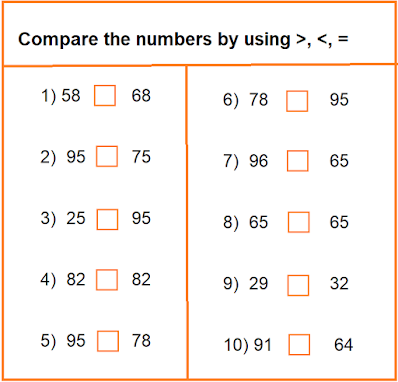# Maths Homework for Class 2 for April 11: Worksheet on Greater than, Lower than and equal to

## Maths Homework for Class 2 for April 11: Worksheet on Greater than, Lower than and equal to

Homework Self Study Mantra provides daily homework and worksheet for the students of Class 1 to 5 and also provides solution for these homework and worksheet. In continuation of this series, here is maths worksheet for class 2 students for April 11.

Let's do it.

## Class 2 maths worksheet.

### A) Compare the numbers by using : >, <, or =.Maths worksheet for class 2

## Maths worksheet for Class 2.

### B) Addition for Class 2 Students.

1)  458 + 25 = ?

2)  658 + 35 = ?

3 ) 365 + 36 = ?

4 )  695 + 52 = ?

Maths worksheet for Class 2